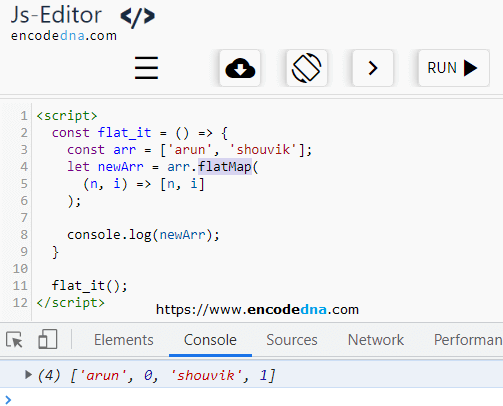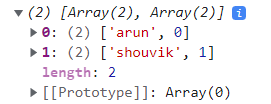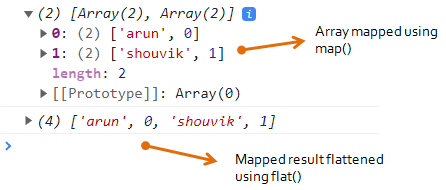﻿ JavaScript array.flatMap() Method with example

# JavaScript array.flatMap() Method with example

The JavaScript array.flatMap() method is a combination of flat() and map() methods. The method first maps each element in an array using the map() method and then flattens it into a new array using the flat() method.

So, instead of calling the 2 methods map() and flat() seperately, we can just use 1 method, the flatMap() method.First, let us see the map() method.

```<script>
const arr = ['arun', 'shouvik'];
let newArr = arr.map(
(n, i) => [n, i]		// where, n is name and i is index.
);

console.log(newArr);      // Output: arun,0,shouvik,1
</script>```

imageNow lets flatten the above result using flat() method.

```<script>
const arr = ['arun', 'shouvik'];
let newArr = arr.map(
(n, i) => [n, i]    // where, n is name and i is index.
);

console.log(newArr);
console.log(newArr.flat());	    // flatten the map() result.

// Output:
// (2) [Array(2), Array(2)]
// (4) ['arun', 0, 'shouvik', 1]
</script>```

imageThe flatMap() is a combination of the above two methods. It shortens the above methods.
See the example. 👇

```<script>
const flat_it = () => {
const arr = ['arun', 'shouvik'];
let newArr = arr.flatMap(
(n, i) => [n, i]
);

console.log(newArr);
}

flat_it();
</script>```

## Filter only even numbers using flatMap() method

Here's another example, where I am filtering only even numbers using the flatMap() method.

```<script>
const even_numbers = () => {
let arr_numbers = [11, 51, 44, 7, 6];
let arr_flattened = arr_numbers.flatMap(
x => x % 2 === 0 ? [x] : []		// return only "even" numbers.
)

console.log(arr_flattened);
}

even_numbers();
</script>```

## flatMap() flattens 1 level

The flatMap() method flattens only 1 level deep. Now, what does this mean. Let us see the above example (even numbers) again. But instead, I have a nested array.

```  let arr_numbers = [11, 51, [44, 7], 6];
let arr_flattened = arr_numbers.flatMap(
x => x % 2 === 0 ? [x] : []		// return only even numbers.
)

console.log(arr_flattened);       // Output: 
```

See values 44 and 7 inside the brackets in the above array. Even though there are two even numbers in the array, the flatMap() however flattens and returns only 1 number, the value 6. It couldn't check number 44.